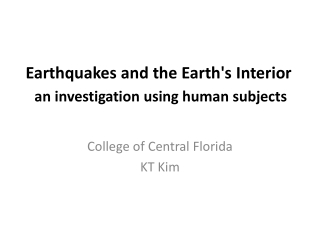DownloadDownload PresentationEarthquakes and the Earth's Interior an investigation using human subjects

# Earthquakes and the Earth's Interior an investigation using human subjects

Télécharger la présentation## Earthquakes and the Earth's Interior an investigation using human subjects

- - - - - - - - - - - - - - - - - - - - - - - - - - - E N D - - - - - - - - - - - - - - - - - - - - - - - - - - -
##### Presentation Transcript

1. Earthquakes and the Earth's Interioran investigation using human subjects College of Central Florida KT Kim

2. Earthquake • Seismology – study of earthquakes and Earth’s interior using seismic waves

3. Earthquake • Stress (Force) causes rock to deform • Three types of deformation • Elastic deformation (Vibration, wave propagation) • Plastic deformation (Folds) • Fracturing (Faults)

4. Earthquake • Earthquake – sudden motion or trembling caused by the abrupt release of energy • Slippage: minor movement (aseismic, fault creep) • Fracture: larger movement (seismic)

5. Earthquake • Waves propagate through medium • Focus – rupture point where energy is released • Epicenter – point on Earth’s surface above the focus

6. Seismic Waves • Body waves – travel through Earth’s interior • P wave – compressional elastic wave pressure wave, primary wave • S wave – shear wave, secondary wave • Surface waves – travel through Earth’s surface • Rayleigh waves – rolling (retrogressive) waves • Love waves – Side-to-side waves

7. Seismic Waves • Measuring seismic waves • Seismograph – the instrument • Seismogram – the record it makes • Measurement of earthquake strength • Mercalli scale – measures damage • Richter scale – measures energy • Moment-magnitude – measures energy as a function of movement and fault surface area

8. Richter Earthquake Magnitude • Measure S-P time (25 seconds) • Measure the largest amplitude (20 mm) • Plot them on the corresponding axes. • Connect a line. • Read a magnitude (5)

9. Example 1 • If we compare two earthquakes; one (A) has a magnitude of 5 and the other (B) has a magnitude of 6. What is an amplitude ratio? • Magnitude difference = 6 – 5 = 1 • Amplitude ratio = 101 • Earthquake B has a 10 times bigger amplitude

10. Example 2 • If we compare two earthquakes; one (A) has a magnitude of 4.5 and the other (B) has a magnitude of 6.5 What is an amplitude ratio? • Magnitude difference = 6.5 – 4.5 = 2 • Amplitude ratio = 102 • Earthquake B has a 100 times bigger amplitude

11. Example 3 • If we compare two earthquakes; one (A) has a magnitude of 3.7 and the other (B) has a magnitude of 6.7 What is an amplitude ratio? • Magnitude difference = 6.7 – 3.7 = 3 • Amplitude ratio = 103 • Earthquake B has a 1000 times bigger amplitude

12. Example 4 • If we compare two earthquakes; one (A) has a magnitude of 4.3 and the other (B) has a magnitude of 6.7 What is an amplitude ratio? • Magnitude difference = 6.7 – 4.3 = 2.4 • Amplitude ratio = 102.4 • Earthquake B has a ~251 times bigger amplitude

13. Locating Earthquakes • P and S waves travel at different speeds • Allows calculation of distance • Time-travel curve • Distance from multiple observations finds a location • Three seismographs

14. Earthquakes & Tectonic Plates • Where do earthquakes occur? • Convergent boundaries • Divergent boundaries • Transform fault boundaries • Plate interiors

15. Earthquakes & Tectonic Plates • Convergent boundaries • One plate sliding under another • Benioff zone • Friction along the down-plunging contact zone

16. Earthquakes & Tectonic Plates • Divergent boundaries • Friction along sliding blocks • Mainly shallow

17. Earthquakes & Tectonic Plates • Transform boundaries • Offsets ridge system • San Andreas fault zone • Strike-slip fault • Fault is vertical • Plate motion along the line of the fault • Fault creep

18. Earthquakes & Tectonic Plates • Plate interiors - infrequent • 1811~12 in New Mardrid, MO • Area is still seeing deformation

19. Earthquake Hazard & Mitigation • Rock and soil – varying responses • Bedrock • Soil type • Topography • Liquefaction • Soil water content • Water table

20. Earthquake Hazard & Mitigation • Construction design and earthquake damage • Regulation of location and materials • Framing materials • Effects of affluence

21. Earthquake Hazard Map

22. Tsunami • Seismic sea wave • Undersea fault motion • Far-traveling wave • Coastal hazard • Sumatra earthquake (2004) • Tohoku earthquake in Japan (2011)

23. Earthquake Prediction • Long-term prediction • Tells where earthquakes are likely to occur • Short-term prediction • Place and Time • Foreshocks • Aftershocks • Monitoring • China, Japan

24. Earth’s Interior • Wave behavior • In homogeneous media, wave propagate equally in all directions • Velocity depends on the nature of material waves are traveling through • Waves refract (bend) when moving from one material to another

25. Earth’s Interior • Moho discontinuity • The crust-mantle boundary • AndrijaMohorovičić (1909) • Waves arrived at distant earthquakes faster than closer ones (Refraction)

26. Earth’s Interior • Structure of the mantle • 2900 km think • 660 discontinuity • 80 % of Earth’s volume

27. Earth’s Interior • Discovery of the core • A shadow zone of seismic waves • S wave does not propagate through the liquid medium  outer core is liquid.